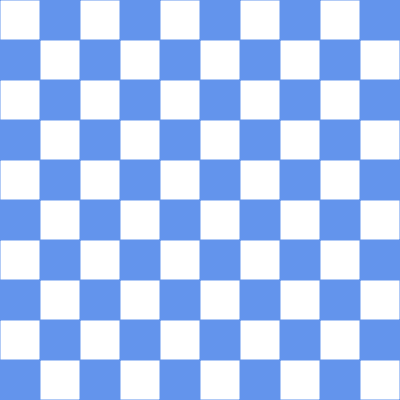# teaching machines

## Sliding Checkerboard

October 27, 2020 by . Filed under public, twoville.

There are a lot of amazing animator-geometrician-illusionists on Twitter, and I want to be like them. But I can’t figure out how they have the time to make what they make. I comfort myself by assuming that they have no friends and no job.

Despite having heaps of friends and a job, I did manage to build an animation the other day. Here it is:The animation was coded in Twoville, a little programming language I’ve been working on to facilitate algorithmic generation of vector graphics. Since I’m really the only one that programs in Twoville right now, I don’t expect you to appreciate the brevity of the code. But I do.



step = 20

with export
size = [512, 512]

with time
start = 0
stop = step * 4
delay = 0.02

for r in -1..11
for c in -1..11
if abs(r) % 2 != abs(c) % 2
// Bottom right
with polygon()
stroke.color = :cornflower
stroke.size = 0.25
color = :cornflower
vertex().position = [c, r] * 10
vertex().position = [c + 1, r] * 10
vertex().position = [c + 1, r + 1] * 10
with translate()
0 -> step * 1 -> t
offset = :zero2
t -> step * 2 -> step * 3 -> t
offset = -[5, ~]
t -> step * 4
offset = -[10, ~]

// Top left
with polygon()
stroke.color = :cornflower
stroke.size = 0.25
color = :cornflower
vertex().position = [c, r] * 10
vertex().position = [c + 1, r + 1] * 10
vertex().position = [c, r + 1] * 10
with translate()
0 -> step * 1 -> t
offset = :zero2
t -> step * 2 -> step * 3 -> t
offset = [5, ~]
t -> step * 4
offset = [10, ~]

var twovilleDiv = jQuery('#twoville_slide_diagonal');
twovilleDiv.closest('pre').replaceWith(twovilleDiv);
document.getElementById('twoville_form_slide_diagonal').submit();

step = 20

with export
size = [512, 512]

with time
start = 0
stop = step * 4
delay = 0.02

for r in -1..11
for c in -1..11
if abs(r) % 2 != abs(c) % 2
// Bottom right
with polygon()
stroke.color = :cornflower
stroke.size = 0.25
color = :cornflower
vertex().position = [c, r] * 10
vertex().position = [c + 1, r] * 10
vertex().position = [c + 1, r + 1] * 10
with translate()
0 -> step * 1 -> t
offset = :zero2
t -> step * 2 -> step * 3 -> t
offset = -[5, ~]
t -> step * 4
offset = -[10, ~]

// Top left
with polygon()
stroke.color = :cornflower
stroke.size = 0.25
color = :cornflower
vertex().position = [c, r] * 10
vertex().position = [c + 1, r + 1] * 10
vertex().position = [c, r + 1] * 10
with translate()
0 -> step * 1 -> t
offset = :zero2
t -> step * 2 -> step * 3 -> t
offset = [5, ~]
t -> step * 4
offset = [10, ~]